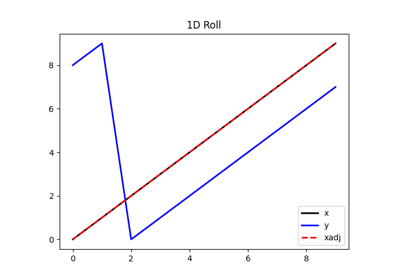# pylops.Roll¶

class pylops.Roll(N, dims=None, dir=0, shift=1, dtype='float64')[source]

Roll along an axis.

Roll a multi-dimensional array along a specified direction dir for a chosen number of samples (shift).

Parameters: N : int Number of samples in model. dims : list, optional Number of samples for each dimension (None if only one dimension is available) dir : int, optional Direction along which rolling is applied. shift : int, optional Number of samples by which elements are shifted dtype : str, optional Type of elements in input array.

Notes

The Roll operator is a thin wrapper around numpy.roll and shifts elements in a multi-dimensional array along a specified direction for a chosen number of samples.

Attributes: shape : tuple Operator shape explicit : bool Operator contains a matrix that can be solved explicitly (True) or not (False)

Methods

 __init__(N[, dims, dir, shift, dtype]) Initialize this LinearOperator. adjoint() Hermitian adjoint. apply_columns(cols) Apply subset of columns of operator cond([uselobpcg]) Condition number of linear operator. conj() Complex conjugate operator div(y[, niter, densesolver]) Solve the linear problem $$\mathbf{y}=\mathbf{A}\mathbf{x}$$. dot(x) Matrix-matrix or matrix-vector multiplication. eigs([neigs, symmetric, niter, uselobpcg]) Most significant eigenvalues of linear operator. matmat(X) Matrix-matrix multiplication. matvec(x) Matrix-vector multiplication. rmatmat(X) Matrix-matrix multiplication. rmatvec(x) Adjoint matrix-vector multiplication. todense([backend]) Return dense matrix. toimag([forw, adj]) Imag operator toreal([forw, adj]) Real operator tosparse() Return sparse matrix. trace([neval, method, backend]) Trace of linear operator. transpose() Transpose this linear operator.

## Examples using pylops.Roll¶Roll